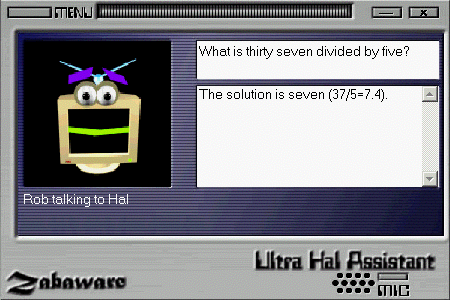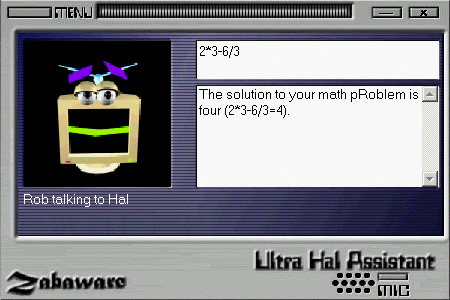Using Hal's Natural Language Calculator Previous   Next

How To Use Hal's Natural Language CalculatorUsing Hal's calculator is easy. Just enter a simple math problem in either words or numbers and Hal will give you the answer. Hal can add, subtract, multiply, divide, calculate square roots, and powers.